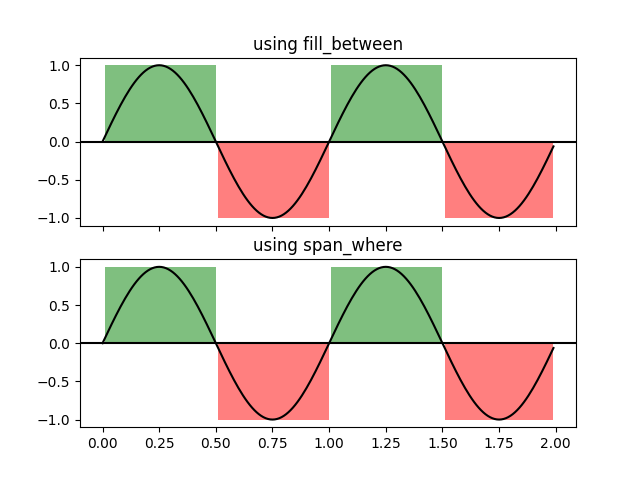# Shade regions defined by a logical mask using fill_between or span_where#

Shade regions where a logical mask is True with Axes.fill_between or with matplotlib.collections.BrokenBarHCollection.span_where.

import numpy as np
import matplotlib.pyplot as plt
import matplotlib.collections as collections

t = np.arange(0.0, 2, 0.01)
s = np.sin(2*np.pi*t)

fig, axs = plt.subplots(2, sharex=True, sharey=True)
for ax in axs:
ax.plot(t, s, color='black')
ax.axhline(0, color='black')

axs.set_title('using fill_between')
axs.fill_between(t, 1, where=s > 0, facecolor='green', alpha=.5)
axs.fill_between(t, -1, where=s < 0, facecolor='red', alpha=.5)

axs.set_title('using span_where')